## 第38届全国物理预赛

•  2022/8/12
•  41 次
•  906 K

1．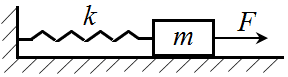如图所示，一轻弹簧左端固定，右端连接一物块。弹簧的劲度系数为 k，物块质量为 m，物块与桌面之间的滑动摩擦因数为 μ。重力加速度大小为 g。现以恒力 FF > μmg）将物块自平衡位置开始向右拉动，则系统的最大势能为

A．$$\frac{1}{{2k}}$$F2              B．$$\frac{2}{{k}}$$F2        C．$$\frac{1}{{2k}}$$(Fμmg)2         D．$$\frac{2}{{k}}$$ (Fμmg)2

【答案】

D

2．L 型的细铜棒在磁感应强度为 B 的匀强磁场中运动，已知 L 型棒两边相互垂直，长度均为 l，运动速度大小均为 v，则铜棒两端电势差的最大值为

A．$$\frac{1}{2}$$Blv              B．Blv                 C．$$\sqrt{2}$$Blv           D．2Blv

【答案】

C

3．氢原子的基态能量为 – 13.6 eV。由一个电子和一个正电子结合成的束缚态（即所谓电子偶素）的基态能量近似为

A．− 1.2 eV          B．− 3.4 eV          C．− 6.8 eV          D．− 27.2 eV

【答案】

C

4．关于放射性元素的半衰期，下列说法正确的是

A．某种放射性元素的半衰期为 T，那么经过时间 T 该放射性元素的原子核个数一定下降为原来的一半。

B．不同种的放射性元素的半衰期长短差别很大，因此同种放射性元素在不同状态下也可能具有不同的半衰期。

C．半衰期是表征放射性元素衰变快慢的物理量，故可通过放射性同位素的衰变来测定时间。

D．若有某种放射性元素的原子 100 个，经过一个半衰期，该种放射性元素的原子个数可能还是 100 个，只是概率很小。

【答案】

CD

5．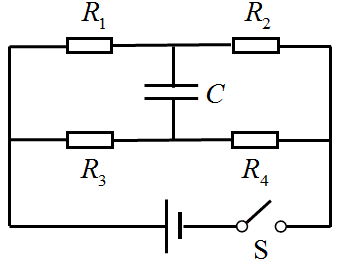在如图所示的电路中，电源电动势为 5 V，内阻不计，R1 = 2 Ω，R2 = 8 Ω，R3 = 4 Ω，R4 = 6 Ω，电容器的电容 C = 10 μF。先将开关 S 闭合，待电路稳定后断开 S，则断开 S 后流经 R4 的电荷量为

A．2 μC        B．3 μC        C．4 μC        D．5 μC

【答案】

B

6．2020 年 11 月 24 日，嫦娥五号发射升空，成为我国首个从月球采样返回的航天器。已知月球质量为 7.4×1022 kg，月球半径为 1.7×103 km，引力常量为 6.67×10−11 N·m2/kg2，该探测器从月球起飞的第一宇宙速度为___________，第二宇宙速度为___________。（结果保留两位有效数字）。

【答案】

1.7 km/s ，2.4 km/s

7．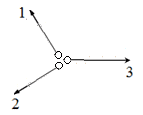一粒水银珠竖直地掉在光滑的水平玻璃板上，分成三粒小水银珠 1、2、3，以相等的速率沿三个方向在玻璃板上运动，如图所示。图中，小水银珠 1 与 2、2 与 3、3 与 1 的运动方向之间的夹角分别为 90°、150°、120°。小水银珠 1、2 的质量之比为 m1m2 为__________，小水银珠 2、3 的质量之比为 m2m3 为___________。

【答案】

1∶$$\sqrt 3$$，$$\sqrt 3$$∶2

8．一质量为 m、半径为 R 的均质小球静止在水平桌面上，小球和桌面之间的动摩擦因数为 μ，最大静摩擦力等于滑动摩擦力，重力加速度大小为 g。用一根水平轻杆击打小球，击打位置位于球心所在的水平面上，击打方向正对球心，击打时间极短，小球获得的水平冲量为 P。从击打结束开始始经_______时间后小球开始纯滚动，小球开始纯滚动时的速度为________。已知小球绕球心的转动惯量为 $$\frac{2}{5}$$mR2

【答案】

$$\frac{{2P}}{{7\mu mg}}$$，$$\frac{{5P}}{{7m}}$$

9．气温为 25℃ 时，在体积为 V 的导热容器中装有湿度为 80%、压强为 p（干燥空气与水蒸汽的分压之和）的空气。保持温度不变，当容器体积缓慢压缩为_________V 时开始有水蒸气液化；此时固定容器体积，将温度降低到 0℃ 后，容器内的压强为___________。设 25℃ 和 0℃ 时水的饱和蒸汽压分别为 p1p2，液化后生成的水的体积可忽略。

【答案】

$$\frac{4}{5}$$ V，$$\frac{{273}}{{298}}\left( {\frac{4}{5}p - {p_1}} \right)$$ + p2

10．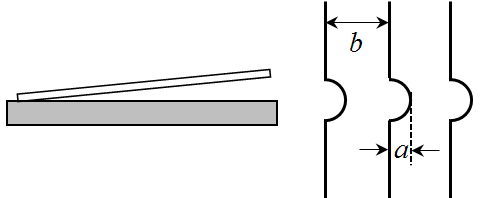等厚干涉常被用来检测工件的表面平整度。如图所示，在经过加工的工件表面放一块薄光学平板玻璃，平板玻璃和工件表面的夹角为 θθ 很小）。用波长为 λ 的单色光垂直照射工件，垂直于工件表面观测时干涉条纹的间距为__________；若观测到如图所示的干涉条纹畸变，则说明工件表面是_______（填“下凹”或“上凸”）的。

【答案】

$$\frac{\lambda }{{2\sin \theta }}$$ 或 $$\frac{\lambda }{{2\theta }}$$ 或 $$\frac{\lambda }{{2\tan \theta }}$$，上凸

11．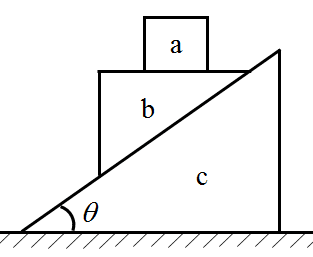如图所示，物块 a、b 叠放在一倾角为 θ 的物块 c的斜面上，物块 c 置于水平地面上。已知物块 a、b、c 的质量均为 m，物块 b 的上表面水平且足够宽，重力加速度大小为 g。不计所有接触面的摩擦。开始时，用外力使 a、b、c 均处于静止状态；撤除该外力后，则在 b 到达斜面 c 的底端之前，各物块运动的加速度以及物块 a、b 之间以及物块 b、c 之间的正压力大小。

【答案】

aa = $$\frac{{4g}}{{4 + {{\cot }^2}\theta }}$$

ac = $$\frac{{2g}}{{4\tan \theta + \cot \theta }}$$

ab = $$\frac{{2g\tan \theta }}{{\sqrt {1 + 4{{\tan }^2}\theta } }}$$

N1 = $$\frac{{mg{{\cot }^2}\theta }}{{4 + {{\cot }^2}\theta }}$$

N2 = $$\frac{{2mg\cos \theta }}{{3{{\sin }^2}\theta + 1}}$$

12．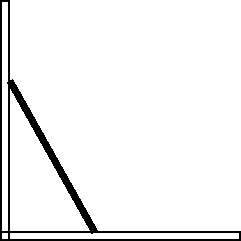如图所示，长度为 L、质量为 m 的均匀金属杆两端靠在垂直于水平面的直角绝缘导轨上，导轨的两臂分别沿水平与竖直方向。初始时刻金属杆静止，与竖直导轨成 30° 角。不计一切摩擦。

（1）试求当杆下滑到与竖直导轨成 60° 角时杆的质心的速度；

（2）假设存在垂直于导轨所在平面（纸面）向里的匀强磁场，磁感应强度大小为 B，求当杆下滑到与竖直导轨成 60° 角时杆两端的感应电动势。

【答案】

（1）vC = $$\sqrt {\frac{{3(\sqrt 3 - 1)}}{8}}$$ gL

（2）ξ = $$\frac{{BL}}{4}\sqrt {\frac{{3(\sqrt 3 - 1)}}{2}gL}$$

13．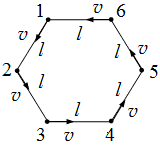6 个小朋友在操场上玩追逐游戏。开始时，6 个小朋友两两间距离相等，构成一正六边形。然后每个小朋友均以不变的速率 v 追赶前面的小朋友（即小朋友 1 追 2、2 追 3、3 追 4、4 追 5、5 追 6、6 追 1），在此过程中，每个小朋友的运动方向总是指向其前方的小朋友。已知某一时刻 t0 = 0，相邻两个小朋友的距离为 l，如图所示。试问：

（1）从 t0 时刻开始，又经过多长时间后面的小朋友可追到前面的小朋友？

（2）从 t0 时刻开始，直至追上前面的小朋友，每个小朋友又跑了多少路程？

（3）在 t0 时刻，每个小朋友的加速度大小是多少？

【答案】

（1）t = $$\frac{{2l}}{v}$$

（2）s = 2l

（3）a = $$\frac{{\sqrt 3 {v^2}}}{{2l}}$$

14．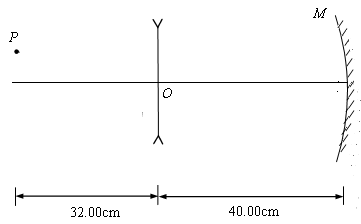如图所示，一薄凹透镜焦距为 – 20.00 cm，一点光源 P 位于该透镜左边并在透镜主轴的正上方，P 在该透镜主轴上的投影距透镜中心 O 点 32.00 cm，P 离光轴距离为 0.30 cm。透镜的右边 40.00 cm 处有一曲率半径为 10.00 cm 的凹面镜，其反射面对着透镜并垂直于主轴放置。试在近轴近似条件下，求最终点光源 P 所成的像点 P′ 相对于 P 点的位置；说明此像是实像还是虚像？

【答案】

15．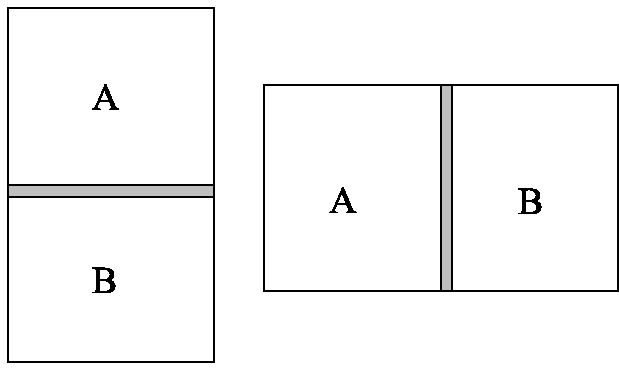如图所示，横截面积为 S 的密闭容器直立在桌面上，容器中有一质量为 M 的活塞将容器分为 A、B 两室，A 室容积为 V1，活塞与器壁之间的摩擦可忽略。A 室中有质量为 m 的氦气，B室中有水蒸气和少量液态水。保持温度不变，将容器缓慢水平放置，B 室中仍有液态水。已知当前温度下水蒸气饱和蒸汽压为 p，水的汽化热为 L，氦气和水蒸气的摩尔质量为 μ1μ2。重力加速度大小为 g。水的体积可忽略。求容器从竖直放置到水平放置的过程中

（1）A 室体积的改变；

（2）B 室从外界吸收的热量。

【答案】

（1）ΔV = $$\frac{{Mg}}{{pS}}$$ V1

（2）Q = $$\left( {\frac{{{\mu _2}}}{{{\mu _1}}} \cdot \frac{{mL}}{{pS - Mg}} + \frac{{{V_1}}}{S}} \right)$$ Mg

16．运算放大器是模拟信号处理的重要元件。其电路元件符号如图 a 所示，理想的运算放大器有正向 +、反向 − 两个输入端和一个输出端，在工作时，运算放大器两个输入端的电势相等，但是并没有电流流入或流出运算放大器。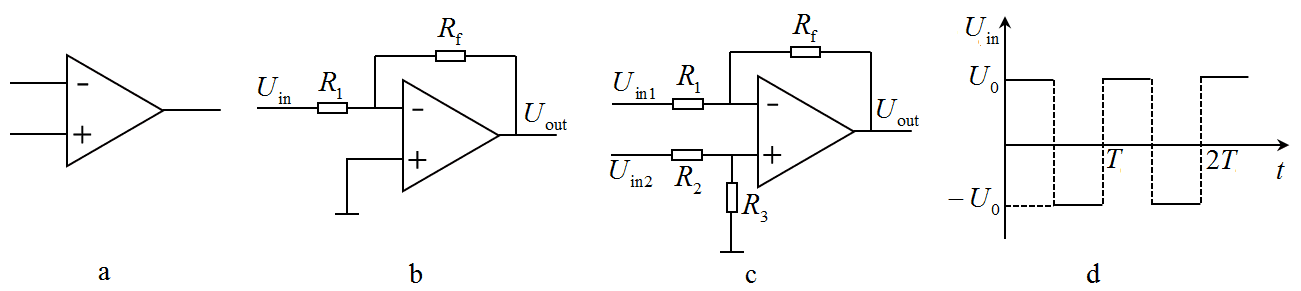（1）若正向输入端接地，试给出如图 b 所示的连接电路的输出电压 Uout 和输入电压 Uin 之间的关系。

（2）如图 c，运算放大器正向和反向输入端均有信号输入。试给出输出电压 Uout 与输入信号 Uin1Uin2 之间的关系；并指出当 R1R2R3Rf 之间满足何种关系时有 Uout = Uin2Uin1

（3）若将（1）中图 b 中的 Rf 换成电容 C，并输入如图 d 所示的方波电压，试画出输出电压 Uout 的波形。

【答案】

（1）Uout = − $$\frac{{{R_f}}}{{{R_1}}}$$Uin

（2）$$\frac{{{R_1}}}{{{R_1} + {R_f}}}$$ = $$\frac{{{R_3}}}{{{R_2} + {R_3}}}$$，$$\frac{{{R_1}}}{{{R_1} + {R_f}}}$$ = $$\frac{{{R_f}}}{{{R_1} + {R_f}}}$$

R1 = RfR2 = R3

（3）如图所示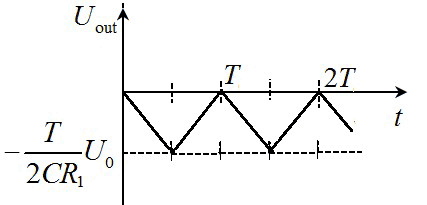2006 - 2023，推荐分辨率1024*768以上，推荐浏览器Chrome、Edge等现代浏览器，截止2021年12月5日的访问次数：1872万9823 站长邮箱